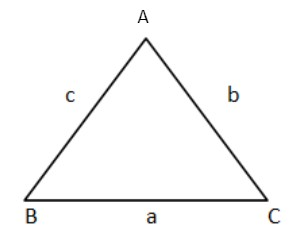QuestionAnswers

# A triangle with one right angle and two acute angles is called :(a) Right angled triangle (b) Acute angled triangle (c) Equilateral triangle (d) None of theseVerified
129k+ views
Hint:Right angled triangle : A triangle in which one angle is ${90^ \circ }$ and the rest of two angles are less that ${90^ \circ }$. Acute angled triangle : A triangle in which all angles are less than ${90^ \circ }$. Equilateral triangle : A triangle in which all the angles are ${60^ \circ }$.Using these definitions we check all the options.

As we know that the triangle is a three sided polygon it contains three angles .
From these three line and angle it is divided further Based on angles it is divided into three types ;Acute angled triangle : The triangle in which all the three angles less than ${90^ \circ }$.
Obtuse angle triangle : The triangle in which one angle is greater than ${90^ \circ }$.
Right angle triangle : The triangle in which one angle is equal to ${90^ \circ }$.${60^ \circ }$
So in the question it is given that the one angle is equal to ${90^ \circ }$ and the other is an acute angle . So from the above theories we know if the one angle of the triangle is equal = ${90^ \circ }$ then it is known by Right angle triangle .

So, the correct answer is “Option A”.

Note:Based on side the triangle is further divided into three types
1) Scalene Triangle : In which all the sides are different in length .
2) Isosceles Triangle : In which the two sides of a triangle are equal and the third side is different in length .
3) Equilateral Triangle : In which all the sides are equal in length.In this triangle all the angles are ${60^ \circ }$.